Скачать презентацию OPTIONS THE TWO BASIC OPTIONS — PUT AND

165c6efc56b5cdf801f7ef89ca125558.ppt

• Количество слайдов: 36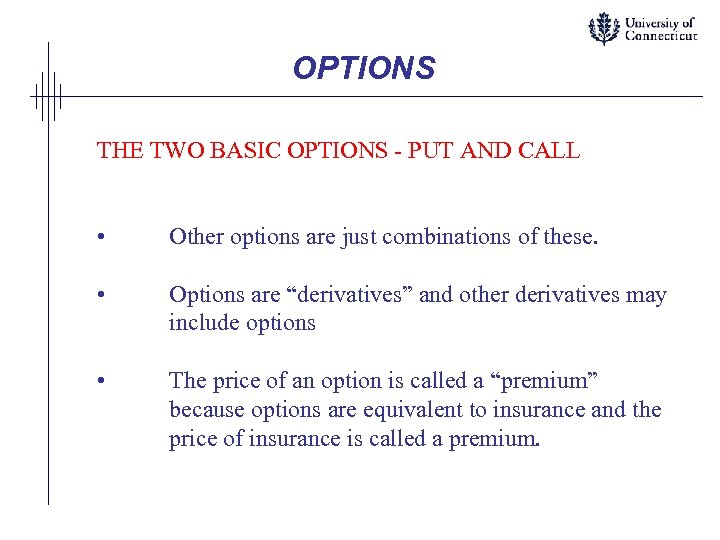OPTIONS THE TWO BASIC OPTIONS - PUT AND CALL • Other options are just combinations of these. • Options are “derivatives” and other derivatives may include options • The price of an option is called a “premium” because options are equivalent to insurance and the price of insurance is called a premium.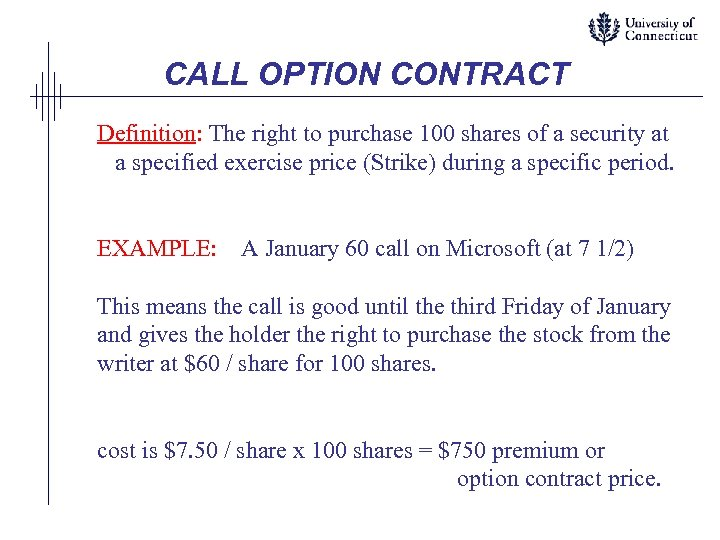CALL OPTION CONTRACT Definition: The right to purchase 100 shares of a security at a specified exercise price (Strike) during a specific period. EXAMPLE: A January 60 call on Microsoft (at 7 1/2) This means the call is good until the third Friday of January and gives the holder the right to purchase the stock from the writer at \$60 / share for 100 shares. cost is \$7. 50 / share x 100 shares = \$750 premium or option contract price.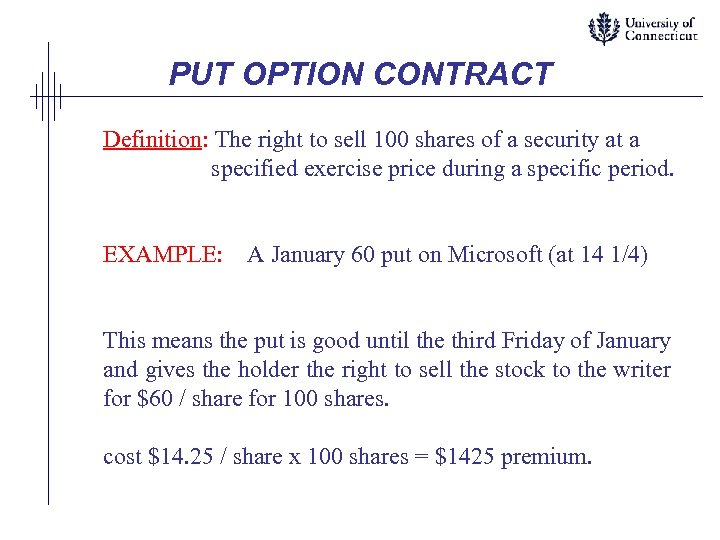PUT OPTION CONTRACT Definition: The right to sell 100 shares of a security at a specified exercise price during a specific period. EXAMPLE: A January 60 put on Microsoft (at 14 1/4) This means the put is good until the third Friday of January and gives the holder the right to sell the stock to the writer for \$60 / share for 100 shares. cost \$14. 25 / share x 100 shares = \$1425 premium.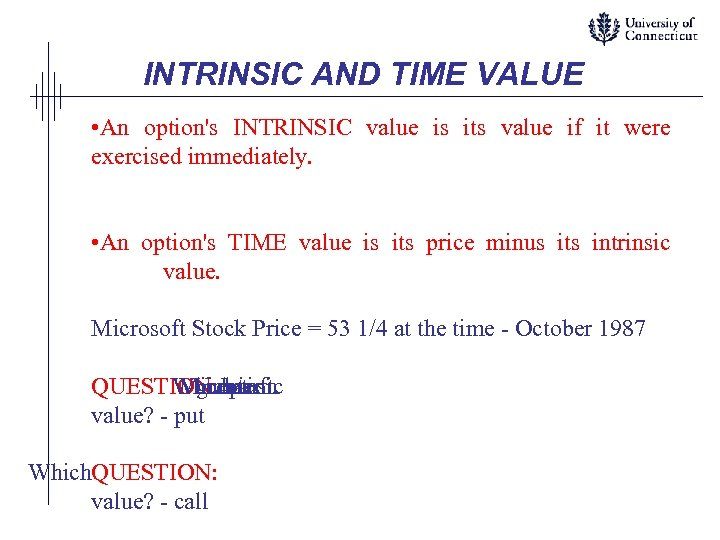INTRINSIC AND TIME VALUE • An option's INTRINSIC value is its value if it were exercised immediately. • An option's TIME value is its price minus its intrinsic value. Microsoft Stock Price = 53 1/4 at the time - October 1987 QUESTION: option Which Microsoft greater intrinsic has value? - put Which. QUESTION: value? - call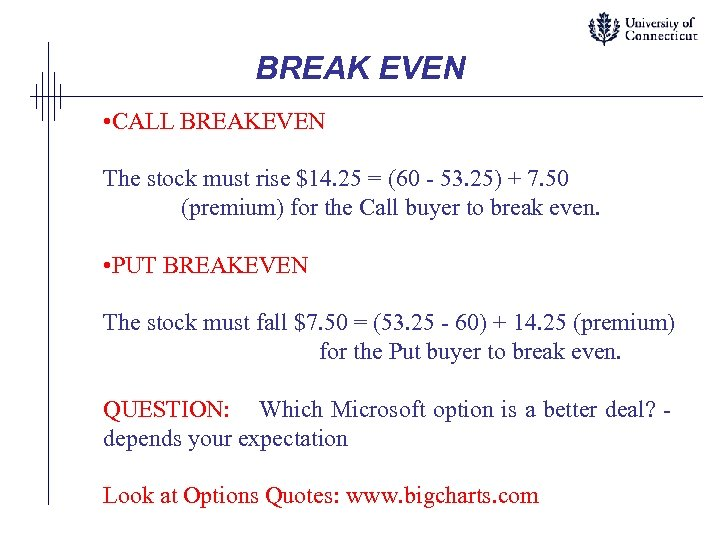BREAK EVEN • CALL BREAKEVEN The stock must rise \$14. 25 = (60 - 53. 25) + 7. 50 (premium) for the Call buyer to break even. • PUT BREAKEVEN The stock must fall \$7. 50 = (53. 25 - 60) + 14. 25 (premium) for the Put buyer to break even. QUESTION: Which Microsoft option is a better deal? depends your expectation Look at Options Quotes: www. bigcharts. com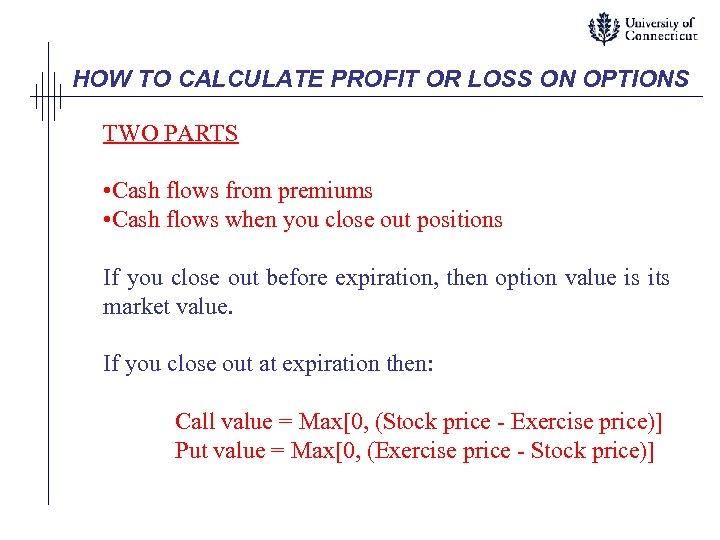HOW TO CALCULATE PROFIT OR LOSS ON OPTIONS TWO PARTS • Cash flows from premiums • Cash flows when you close out positions If you close out before expiration, then option value is its market value. If you close out at expiration then: Call value = Max[0, (Stock price - Exercise price)] Put value = Max[0, (Exercise price - Stock price)]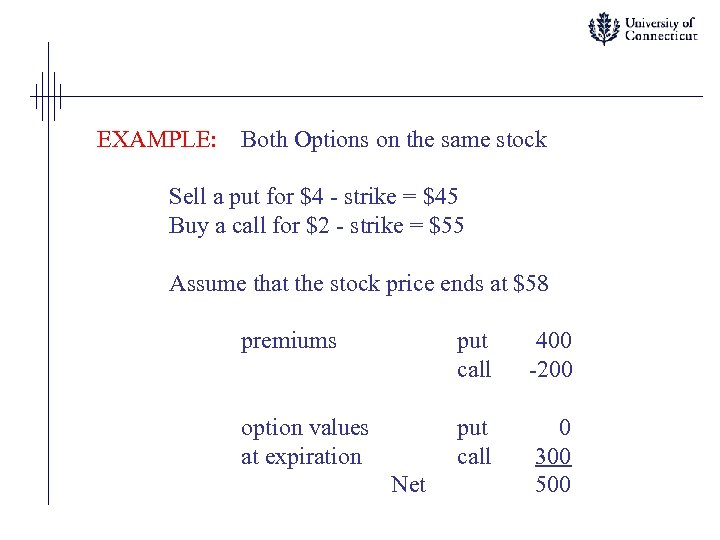EXAMPLE: Both Options on the same stock Sell a put for \$4 - strike = \$45 Buy a call for \$2 - strike = \$55 Assume that the stock price ends at \$58 premiums put call 400 -200 option values at expiration put call 0 300 500 Net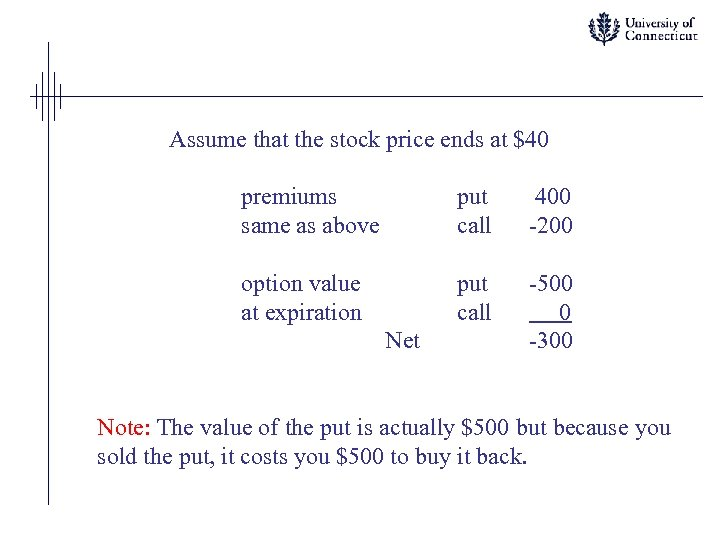Assume that the stock price ends at \$40 premiums same as above put call 400 -200 option value at expiration put call -500 0 -300 Net Note: The value of the put is actually \$500 but because you sold the put, it costs you \$500 to buy it back.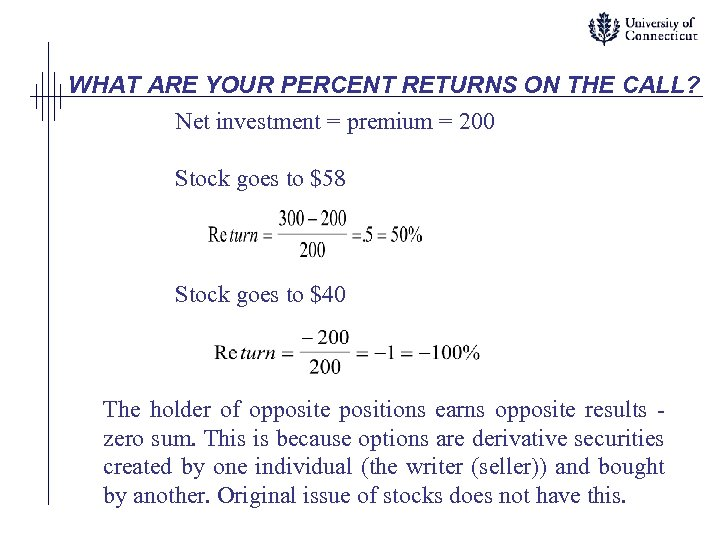WHAT ARE YOUR PERCENT RETURNS ON THE CALL? Net investment = premium = 200 Stock goes to \$58 Stock goes to \$40 The holder of opposite positions earns opposite results zero sum. This is because options are derivative securities created by one individual (the writer (seller)) and bought by another. Original issue of stocks does not have this.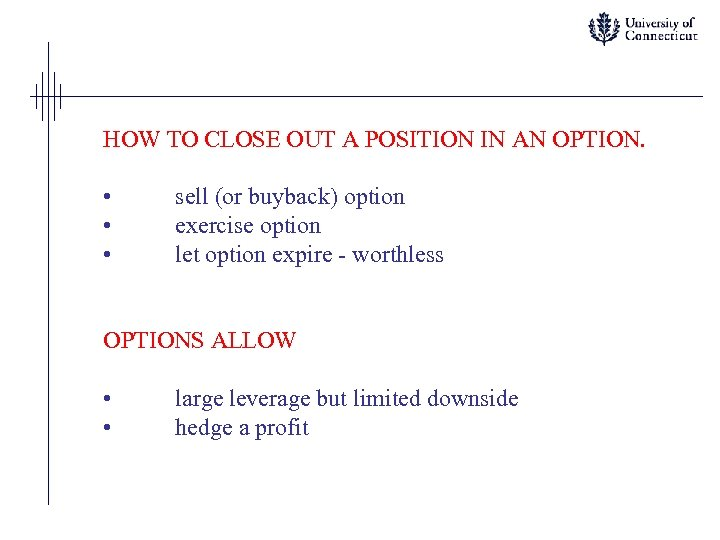HOW TO CLOSE OUT A POSITION IN AN OPTION. • • • sell (or buyback) option exercise option let option expire - worthless OPTIONS ALLOW • • large leverage but limited downside hedge a profit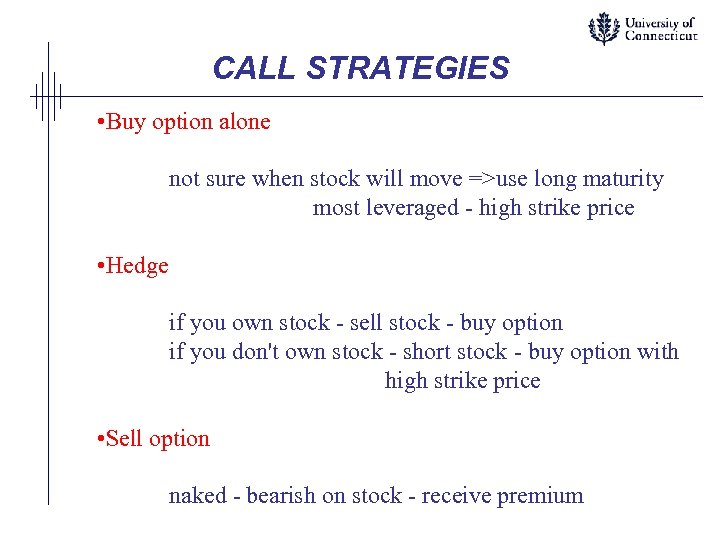CALL STRATEGIES • Buy option alone not sure when stock will move =>use long maturity most leveraged - high strike price • Hedge if you own stock - sell stock - buy option if you don't own stock - short stock - buy option with high strike price • Sell option naked - bearish on stock - receive premium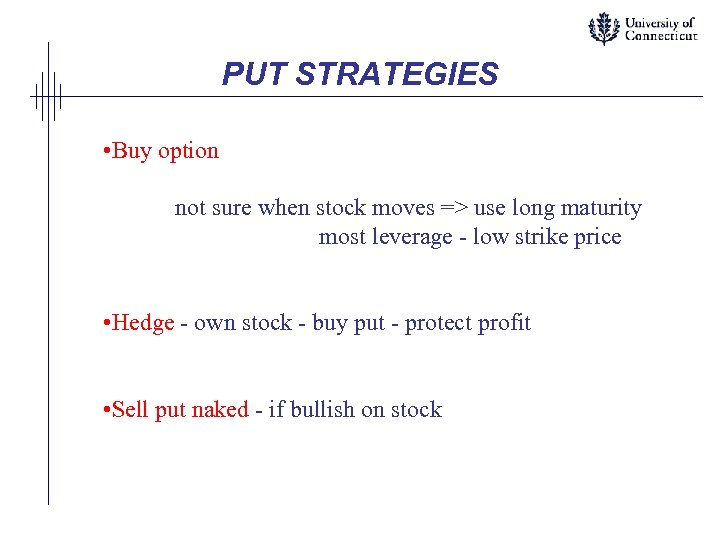PUT STRATEGIES • Buy option not sure when stock moves => use long maturity most leverage - low strike price • Hedge - own stock - buy put - protect profit • Sell put naked - if bullish on stock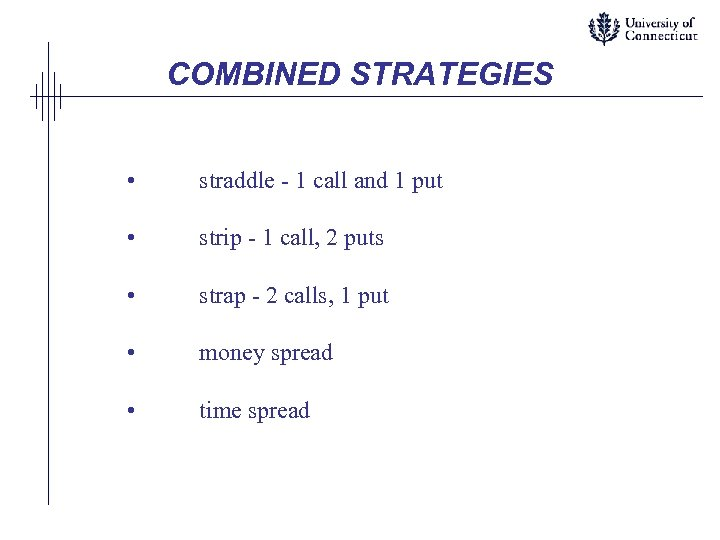COMBINED STRATEGIES • straddle - 1 call and 1 put • strip - 1 call, 2 puts • strap - 2 calls, 1 put • money spread • time spread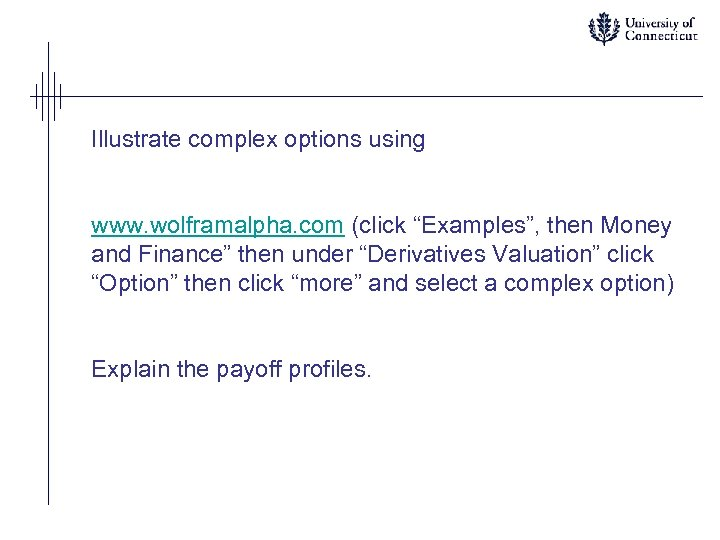Illustrate complex options using www. wolframalpha. com (click “Examples”, then Money and Finance” then under “Derivatives Valuation” click “Option” then click “more” and select a complex option) Explain the payoff profiles.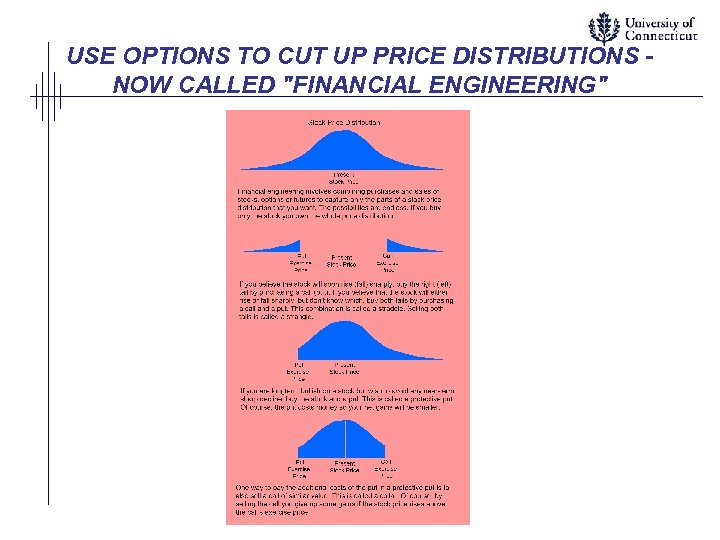USE OPTIONS TO CUT UP PRICE DISTRIBUTIONS NOW CALLED "FINANCIAL ENGINEERING"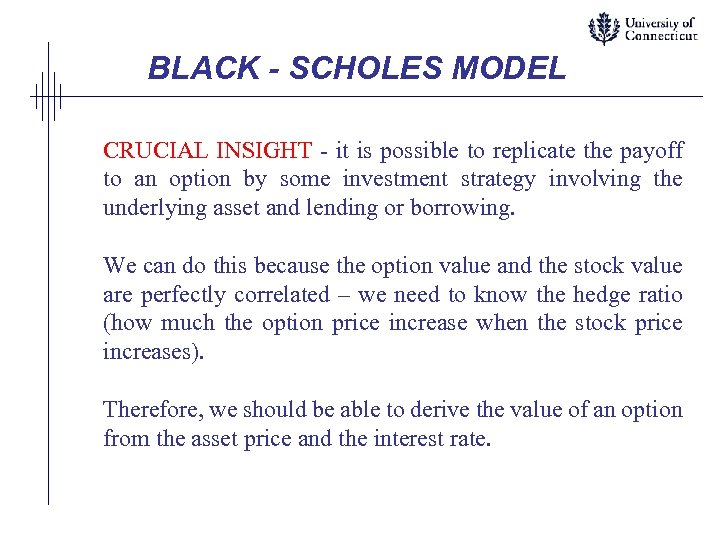BLACK - SCHOLES MODEL CRUCIAL INSIGHT - it is possible to replicate the payoff to an option by some investment strategy involving the underlying asset and lending or borrowing. We can do this because the option value and the stock value are perfectly correlated – we need to know the hedge ratio (how much the option price increase when the stock price increases). Therefore, we should be able to derive the value of an option from the asset price and the interest rate.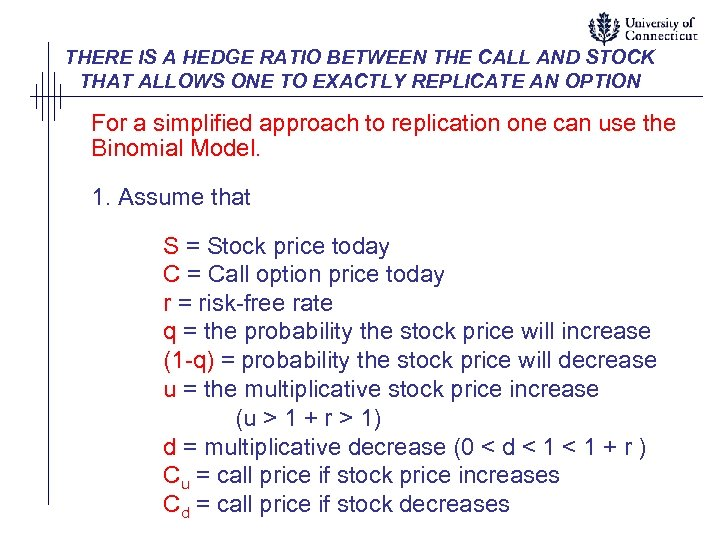THERE IS A HEDGE RATIO BETWEEN THE CALL AND STOCK THAT ALLOWS ONE TO EXACTLY REPLICATE AN OPTION For a simplified approach to replication one can use the Binomial Model. 1. Assume that S = Stock price today C = Call option price today r = risk-free rate q = the probability the stock price will increase (1 -q) = probability the stock price will decrease u = the multiplicative stock price increase (u > 1 + r > 1) d = multiplicative decrease (0 < d < 1 + r ) Cu = call price if stock price increases Cd = call price if stock decreases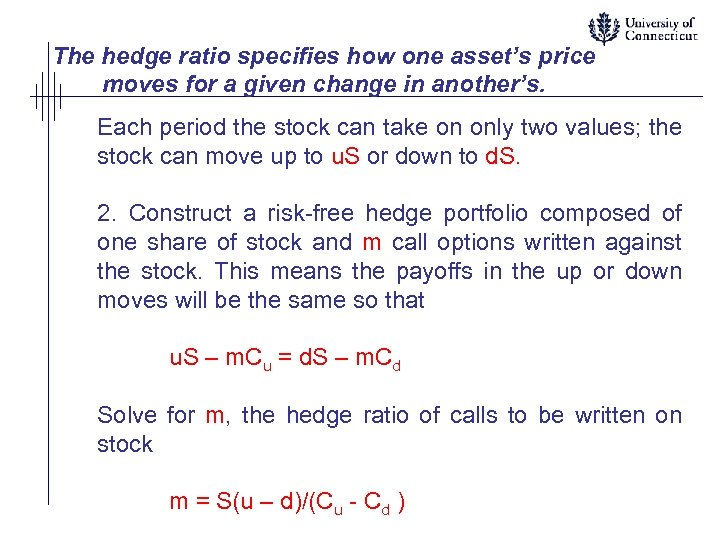The hedge ratio specifies how one asset’s price moves for a given change in another’s. Each period the stock can take on only two values; the stock can move up to u. S or down to d. S. 2. Construct a risk-free hedge portfolio composed of one share of stock and m call options written against the stock. This means the payoffs in the up or down moves will be the same so that u. S – m. Cu = d. S – m. Cd Solve for m, the hedge ratio of calls to be written on stock m = S(u – d)/(Cu - Cd )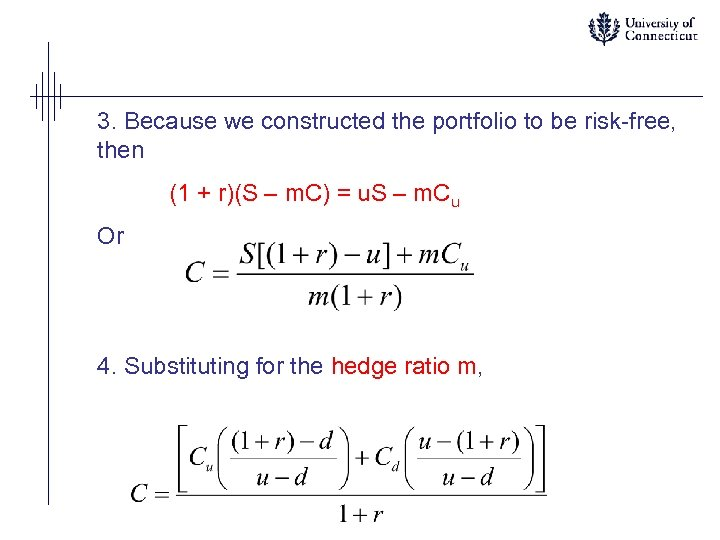3. Because we constructed the portfolio to be risk-free, then (1 + r)(S – m. C) = u. S – m. Cu Or 4. Substituting for the hedge ratio m,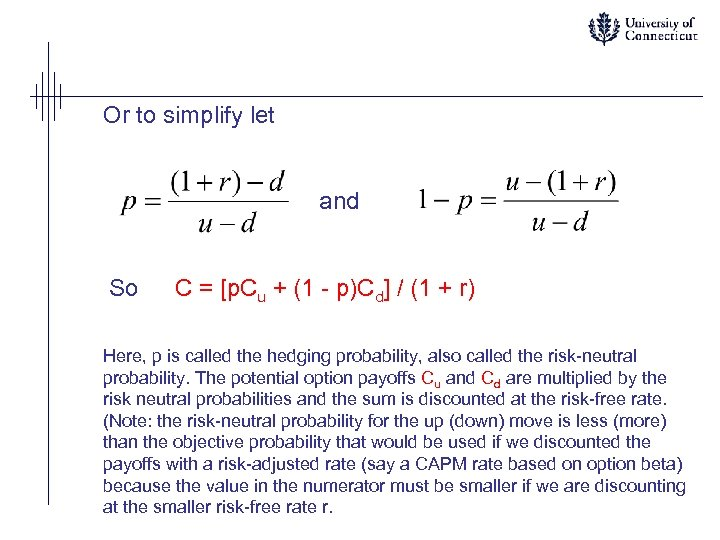Or to simplify let and So C = [p. Cu + (1 - p)Cd] / (1 + r) Here, p is called the hedging probability, also called the risk-neutral probability. The potential option payoffs Cu and Cd are multiplied by the risk neutral probabilities and the sum is discounted at the risk-free rate. (Note: the risk-neutral probability for the up (down) move is less (more) than the objective probability that would be used if we discounted the payoffs with a risk-adjusted rate (say a CAPM rate based on option beta) because the value in the numerator must be smaller if we are discounting at the smaller risk-free rate r.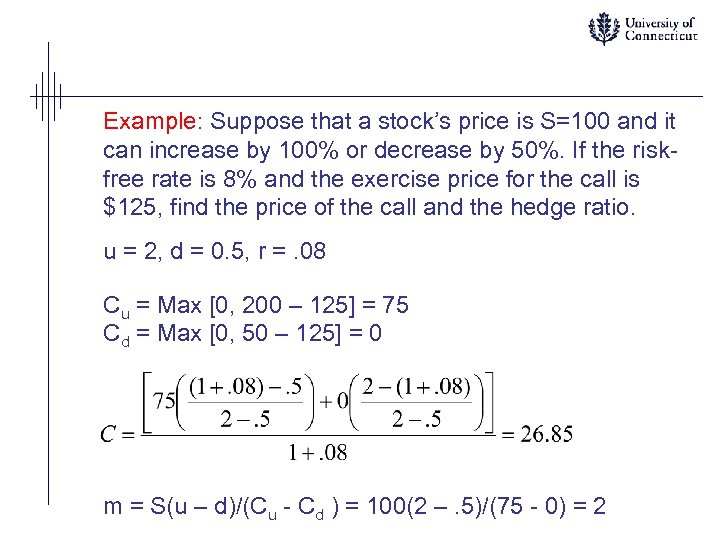Example: Suppose that a stock’s price is S=100 and it can increase by 100% or decrease by 50%. If the riskfree rate is 8% and the exercise price for the call is \$125, find the price of the call and the hedge ratio. u = 2, d = 0. 5, r =. 08 Cu = Max [0, 200 – 125] = 75 Cd = Max [0, 50 – 125] = 0 m = S(u – d)/(Cu - Cd ) = 100(2 –. 5)/(75 - 0) = 2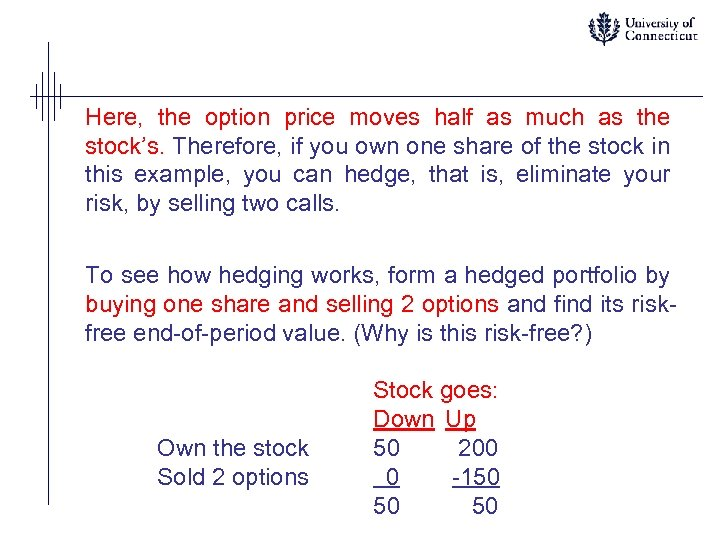Here, the option price moves half as much as the stock’s. Therefore, if you own one share of the stock in this example, you can hedge, that is, eliminate your risk, by selling two calls. To see how hedging works, form a hedged portfolio by buying one share and selling 2 options and find its riskfree end-of-period value. (Why is this risk-free? ) Own the stock Sold 2 options Stock goes: Down Up 50 200 0 -150 50 50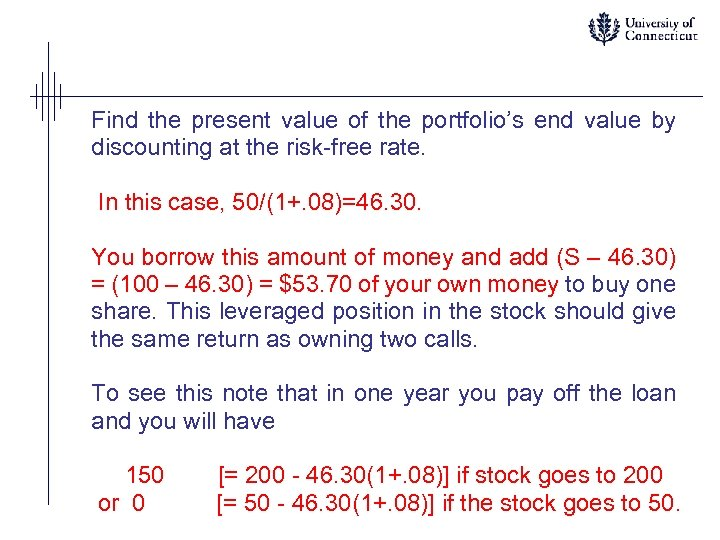Find the present value of the portfolio’s end value by discounting at the risk-free rate. In this case, 50/(1+. 08)=46. 30. You borrow this amount of money and add (S – 46. 30) = (100 – 46. 30) = \$53. 70 of your own money to buy one share. This leveraged position in the stock should give the same return as owning two calls. To see this note that in one year you pay off the loan and you will have 150 or 0 [= 200 - 46. 30(1+. 08)] if stock goes to 200 [= 50 - 46. 30(1+. 08)] if the stock goes to 50.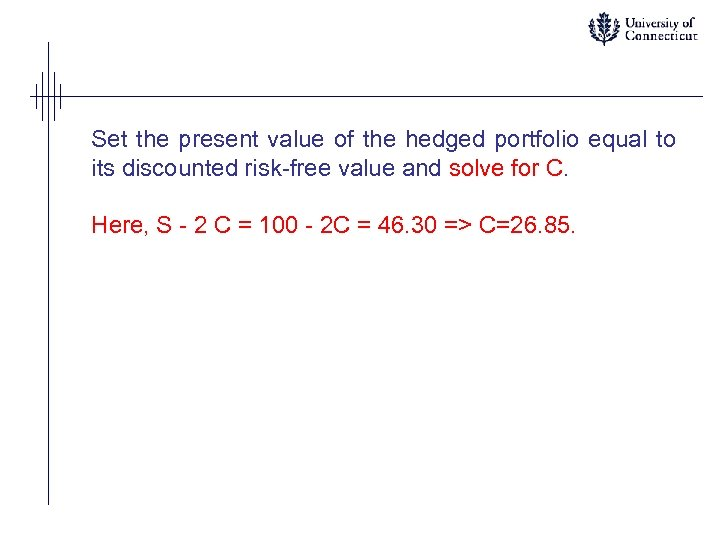Set the present value of the hedged portfolio equal to its discounted risk-free value and solve for C. Here, S - 2 C = 100 - 2 C = 46. 30 => C=26. 85.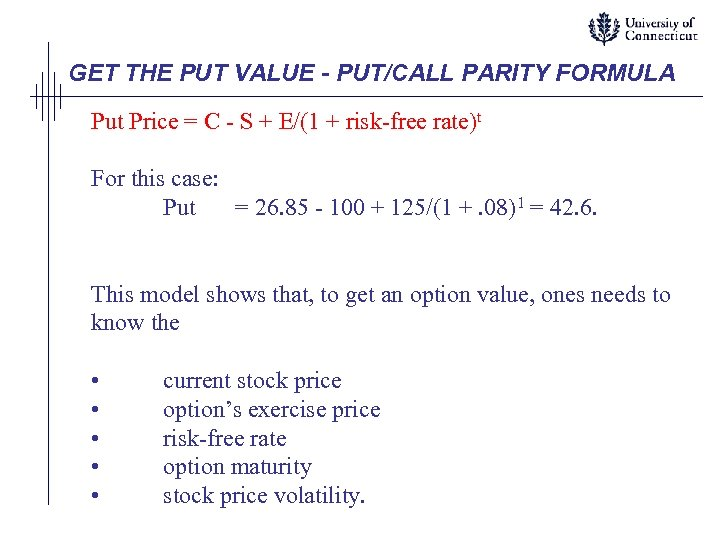GET THE PUT VALUE - PUT/CALL PARITY FORMULA Put Price = C - S + E/(1 + risk-free rate)t For this case: Put = 26. 85 - 100 + 125/(1 +. 08)1 = 42. 6. This model shows that, to get an option value, ones needs to know the • • • current stock price option’s exercise price risk-free rate option maturity stock price volatility.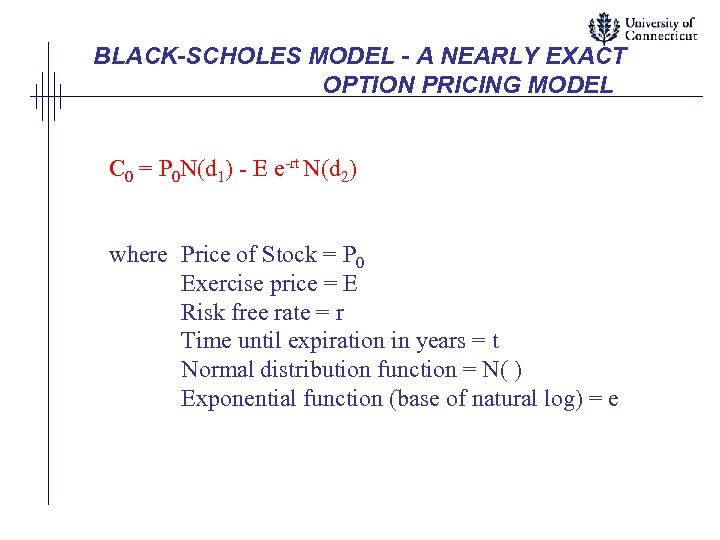BLACK-SCHOLES MODEL - A NEARLY EXACT OPTION PRICING MODEL C 0 = P 0 N(d 1) - E e-rt N(d 2) where Price of Stock = P 0 Exercise price = E Risk free rate = r Time until expiration in years = t Normal distribution function = N( ) Exponential function (base of natural log) = e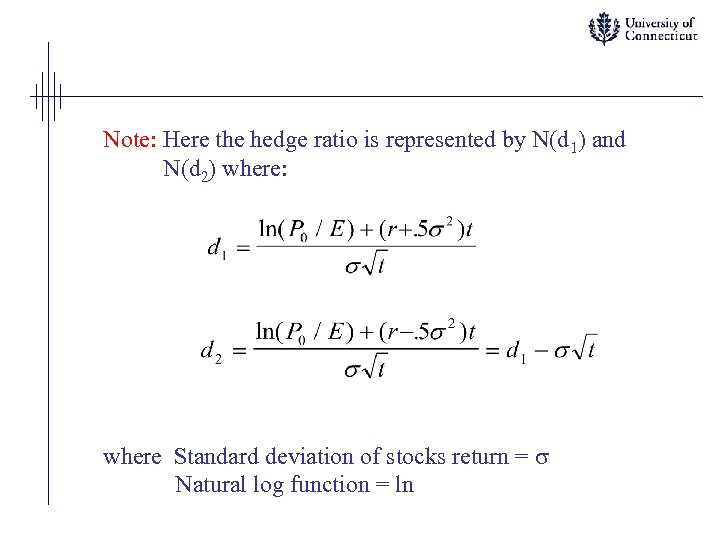Note: Here the hedge ratio is represented by N(d 1) and N(d 2) where: where Standard deviation of stocks return = Natural log function = ln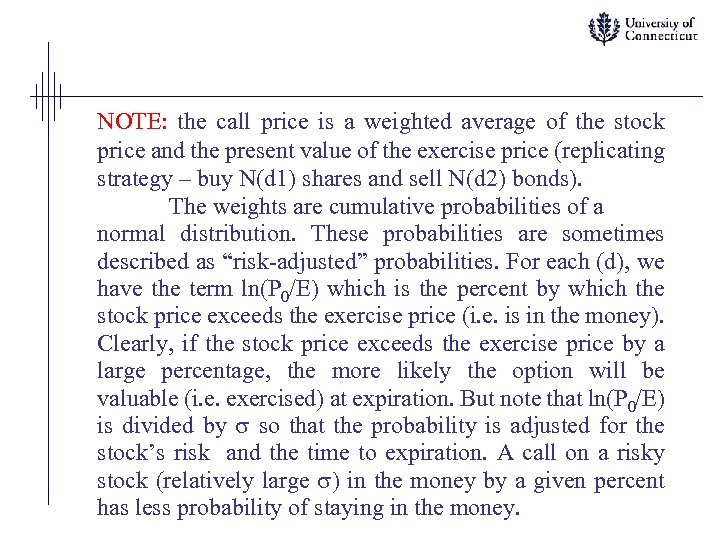NOTE: the call price is a weighted average of the stock price and the present value of the exercise price (replicating strategy – buy N(d 1) shares and sell N(d 2) bonds). The weights are cumulative probabilities of a normal distribution. These probabilities are sometimes described as “risk-adjusted” probabilities. For each (d), we have the term ln(P 0/E) which is the percent by which the stock price exceeds the exercise price (i. e. is in the money). Clearly, if the stock price exceeds the exercise price by a large percentage, the more likely the option will be valuable (i. e. exercised) at expiration. But note that ln(P 0/E) is divided by so that the probability is adjusted for the stock’s risk and the time to expiration. A call on a risky stock (relatively large ) in the money by a given percent has less probability of staying in the money.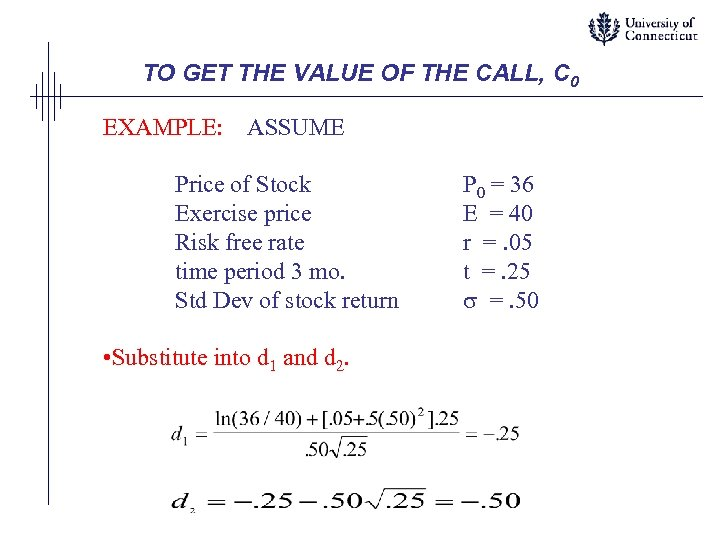TO GET THE VALUE OF THE CALL, C 0 EXAMPLE: ASSUME Price of Stock Exercise price Risk free rate time period 3 mo. Std Dev of stock return • Substitute into d 1 and d 2. P 0 = 36 E = 40 r =. 05 t =. 25 =. 50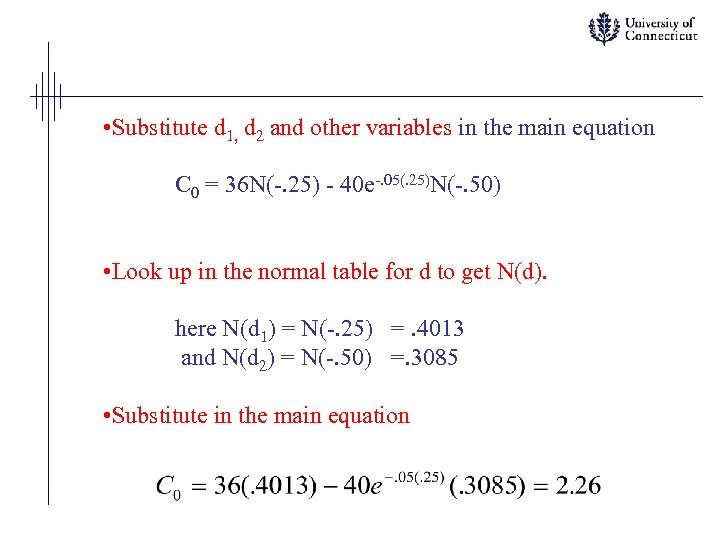• Substitute d 1, d 2 and other variables in the main equation C 0 = 36 N(-. 25) - 40 e-. 05(. 25)N(-. 50) • Look up in the normal table for d to get N(d). here N(d 1) = N(-. 25) =. 4013 and N(d 2) = N(-. 50) =. 3085 • Substitute in the main equation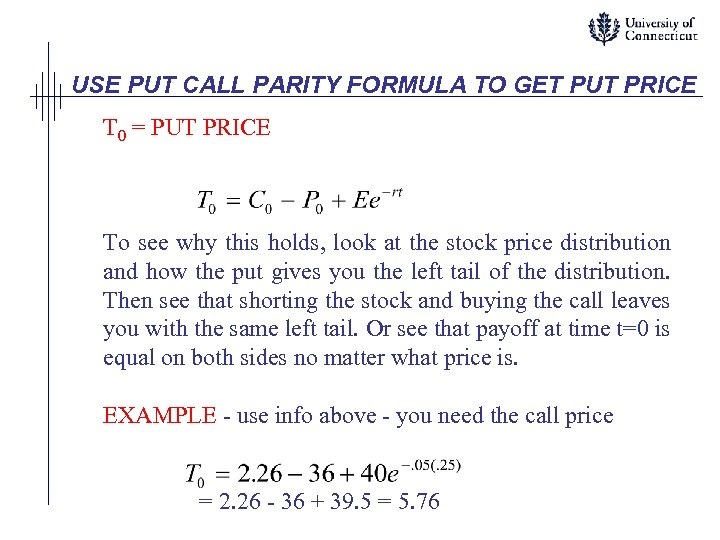USE PUT CALL PARITY FORMULA TO GET PUT PRICE T 0 = PUT PRICE To see why this holds, look at the stock price distribution and how the put gives you the left tail of the distribution. Then see that shorting the stock and buying the call leaves you with the same left tail. Or see that payoff at time t=0 is equal on both sides no matter what price is. EXAMPLE - use info above - you need the call price = 2. 26 - 36 + 39. 5 = 5. 76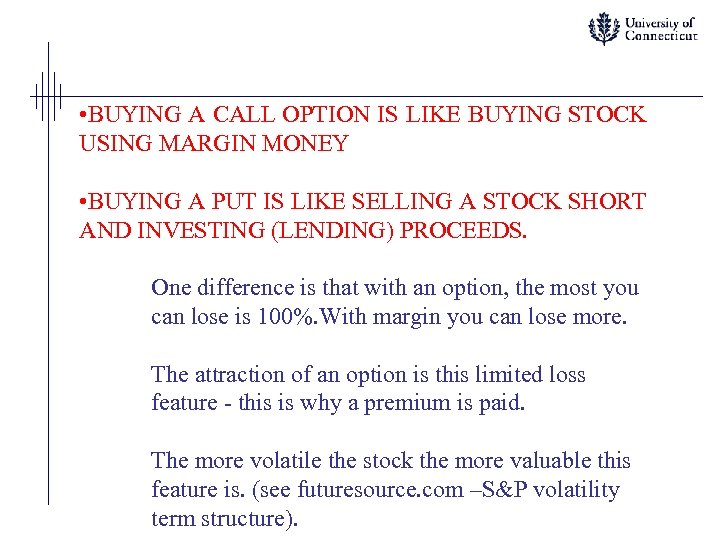• BUYING A CALL OPTION IS LIKE BUYING STOCK USING MARGIN MONEY • BUYING A PUT IS LIKE SELLING A STOCK SHORT AND INVESTING (LENDING) PROCEEDS. One difference is that with an option, the most you can lose is 100%. With margin you can lose more. The attraction of an option is this limited loss feature - this is why a premium is paid. The more volatile the stock the more valuable this feature is. (see futuresource. com –S&P volatility term structure).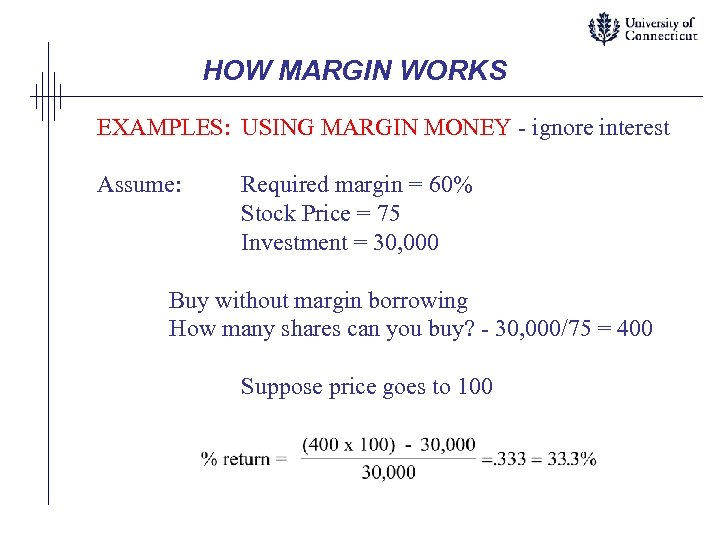HOW MARGIN WORKS EXAMPLES: USING MARGIN MONEY - ignore interest Assume: Required margin = 60% Stock Price = 75 Investment = 30, 000 Buy without margin borrowing How many shares can you buy? - 30, 000/75 = 400 Suppose price goes to 100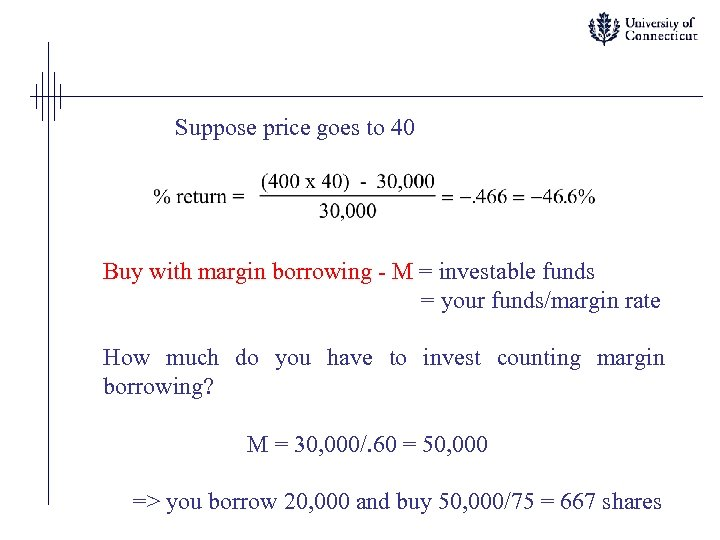Suppose price goes to 40 Buy with margin borrowing - M = investable funds = your funds/margin rate How much do you have to invest counting margin borrowing? M = 30, 000/. 60 = 50, 000 => you borrow 20, 000 and buy 50, 000/75 = 667 shares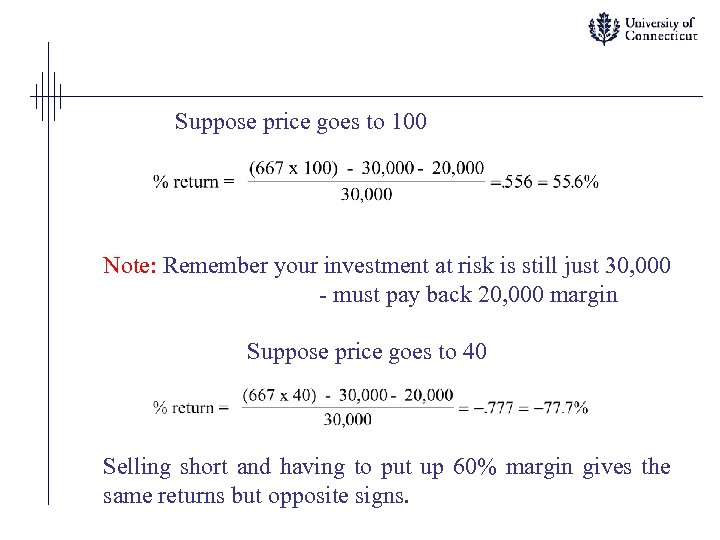Suppose price goes to 100 Note: Remember your investment at risk is still just 30, 000 - must pay back 20, 000 margin Suppose price goes to 40 Selling short and having to put up 60% margin gives the same returns but opposite signs.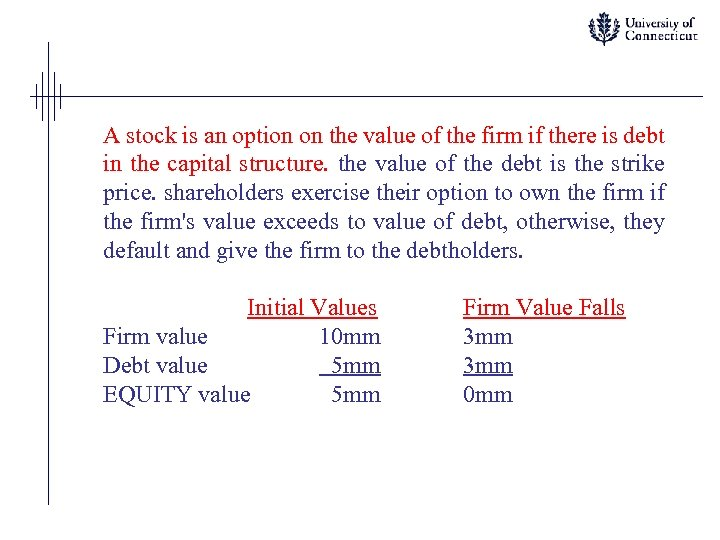A stock is an option on the value of the firm if there is debt in the capital structure. the value of the debt is the strike price. shareholders exercise their option to own the firm if the firm's value exceeds to value of debt, otherwise, they default and give the firm to the debtholders. Initial Values Firm value 10 mm Debt value 5 mm EQUITY value 5 mm Firm Value Falls 3 mm 0 mm